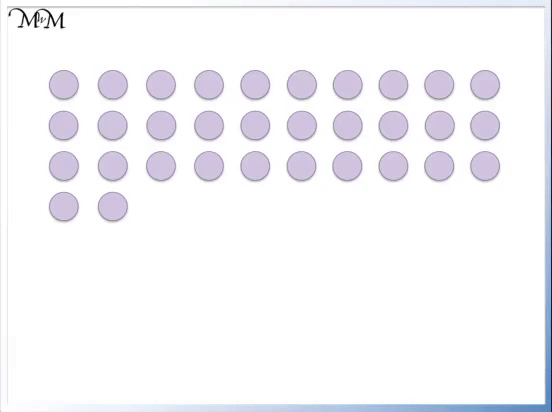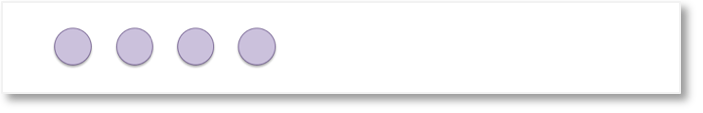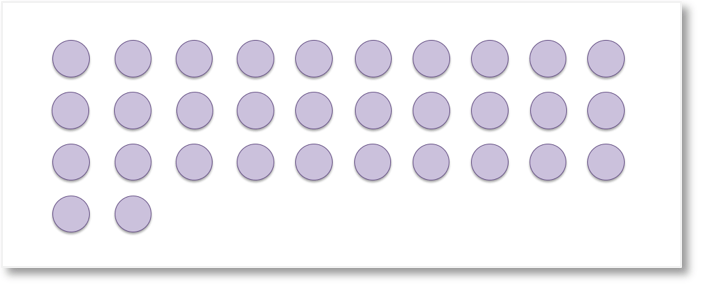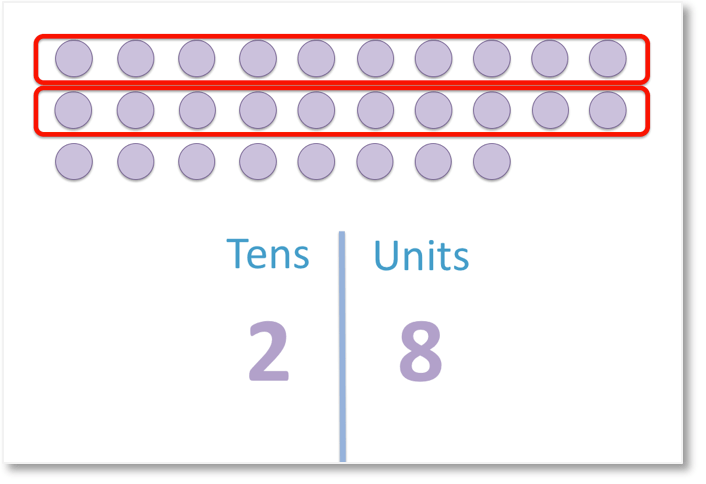# Tens and Units Place Value Chart

Tens and Units Place Value Columns• To help us count the total number of counters we will use a place value chart and collect groups of ten counters together.
• There are 3 groups of ten counters so we write the ‘3’ in the tens column.
• There are 2 counters left over so we write the digit ‘2’ in the units column.
• We write the digits ‘3’ and ‘2’ together as our total two-digit number: 32.

We count groups of ten and then count the single units. The digits written together in place value columns form our total number.• The number 27 is made up of two : ‘2’ and ‘7’.
• The digit ‘7’ is in the units column and represents the value of ‘7’.
• The digit ‘2’ is in the tens column and represents the value of ’20’.
• 27 can be into 20 and 7.# What are Tens and Units (Ones) in Number Place Value Charts?

When we have a small number of counters, it is easy to count how many we have.

We can count up in ones.

We can also say that we count each ‘unit’. Some people say we can count up in ‘ones’ and others will say we count in ‘units’. Both of these words are used in schools when talking about place value.In the example above, we can count 4 counters. We say that have four ones.However, in the example above, it is less easy to immediately see how many counters we have. We will learn how to write the number of counters in a place value chart in this example.To make it easier to count the number of counters, we can group the counters into groups of ten.

Each group of ten counters is shown circled in red. It is now much easier to see how many counters we have in total because we can count the groups of ten and groups of units separately.

We have 3 groups of ten and 2 units.

We can represent the number of counters in a place value chart and this will allow us to write the tens and units as one number.We can show that we have 3 tens by writing the

‘3’ in the tens column.

We can show that we have 2 units by writing the digit of ‘2’ in the units column.

The individual numerals of ‘3’ and ‘2’ are called digits.

Combining the two digits in our place value chart gives us the total number of 32.

Because 32 is made up of two digits: 3 and 2, we say that 32 is a two-digit number.

Below, we have another place value chart example.We can begin by collecting the counters into groups of ten counters.We have 2 groups of ten counters, so we say that we have 2 tens.

We have 8 individual counters left over, so we have 8 units (or 8 ones).This means that we write a 2 in the tens column of our place value chart to show that there are 2 tens.

We write an 8 in the units column of the place value chart to show that we have 8 units.

The two digits of ‘2’ and ‘8’ form our final two-digit number of 28.

The number of counters is therefore 28.

We will now look at how our tens and ones place value columns allow us to

our two-digit numbers into tens and units.

Considering the number ’63’, we can see that it is made up of two digits: ‘6’ and then ‘3’.The digit of ‘3’ is in the units (or ones) column and is just worth 3.

Whereas the digit of ‘6’ is in the tens place value column and is worth 60.This is because 6 tens are 60.

63 can be partitioned into 6 tens and 3 units (or 3 ones).Now try our lesson on Hundreds, Tens and Units where we learn how to extend our knowledge of numbers to 3-digit numbers larger than one hundred.